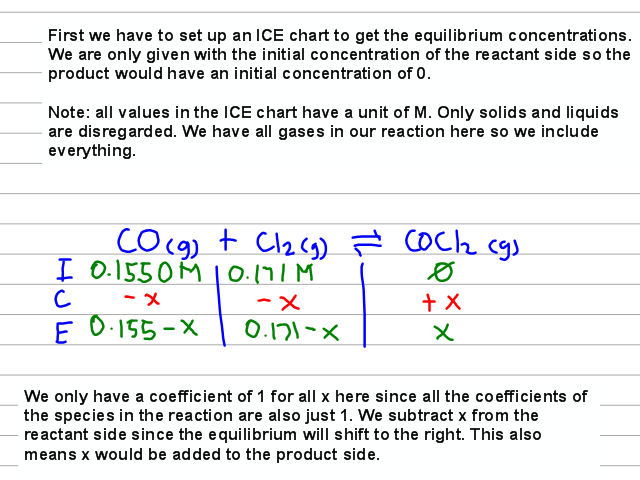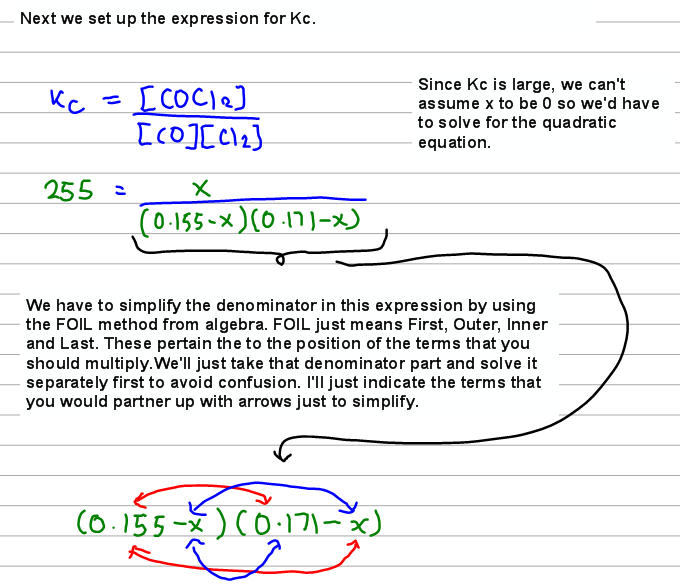# Problem: For the reaction, CO(g) + Cl2(g) ⇌ COCl2(g)       Kc = 255 at 1000 K. If a reaction mixture initially contains a CO concentration of 0.1550 M and a Cl2 concentration of 0.171 M at 1000 K.What is the equilibrium concentration of CO at 1000 K?What is the equilibrium concentration of Cl 2 at 1000 K?What is the equilibrium concentration of COCl2 at 1000 K?

###### FREE Expert Solution
89% (393 ratings)
###### FREE Expert Solution89% (393 ratings)###### Problem Details

For the reaction,

CO(g) + Cl2(g) ⇌ COCl2(g)       Kc = 255 at 1000 K.

If a reaction mixture initially contains a CO concentration of 0.1550 M and a Cl2 concentration of 0.171 M at 1000 K.

What is the equilibrium concentration of CO at 1000 K?

What is the equilibrium concentration of Cl 2 at 1000 K?

What is the equilibrium concentration of COCl2 at 1000 K?

What scientific concept do you need to know in order to solve this problem?

Our tutors have indicated that to solve this problem you will need to apply the ICE Chart concept. You can view video lessons to learn ICE Chart. Or if you need more ICE Chart practice, you can also practice ICE Chart practice problems.

What is the difficulty of this problem?

Our tutors rated the difficulty ofFor the reaction, CO(g) + Cl2(g) ⇌ COCl2(g)       Kc = 255 a...as high difficulty.

How long does this problem take to solve?

Our expert Chemistry tutor, Dasha took 13 minutes and 42 seconds to solve this problem. You can follow their steps in the video explanation above.

What professor is this problem relevant for?

Based on our data, we think this problem is relevant for Professor Billman's class at Abilene Christian University.